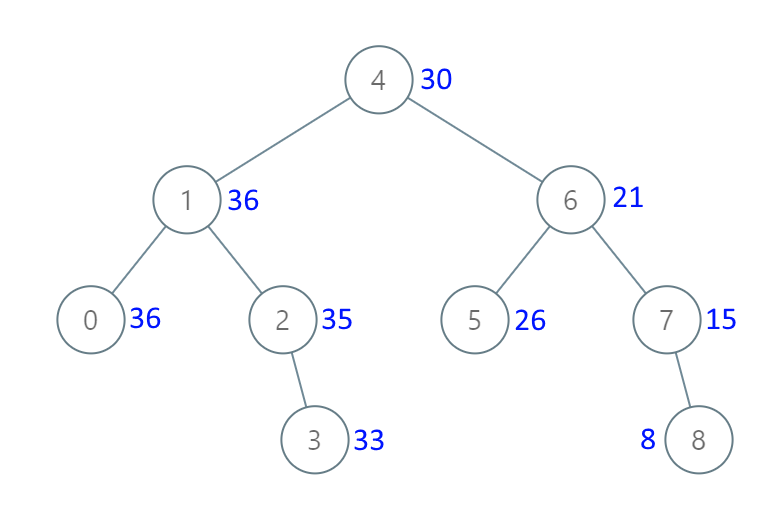### LeetCode• ㊗️
• 大家
• offer
• 多多！

## Problem

Given the root of a Binary Search Tree (BST), convert it to a Greater Tree such that every key of the original BST is changed to the original key plus the sum of all keys greater than the original key in BST.

As a reminder, a binary search tree is a tree that satisfies these constraints:

• The left subtree of a node contains only nodes with keys less than the node’s key.
• The right subtree of a node contains only nodes with keys greater than the node’s key.
• Both the left and right subtrees must also be binary search trees.

Example 1:Input: root = [4,1,6,0,2,5,7,null,null,null,3,null,null,null,8]
Output: [30,36,21,36,35,26,15,null,null,null,33,null,null,null,8]


Example 2:

Input: root = [0,null,1]
Output: [1,null,1]


Constraints:

• The number of nodes in the tree is in the range [0, 10^4].
• -10^4 <= Node.val <= 10^4
• All the values in the tree are unique.
• root is guaranteed to be a valid binary search tree.

Note: This question is the same as 1038

## Code

class Solution {
int sum = 0;

public TreeNode convertBST(TreeNode root) {
if (root == null) return null;

convertBST(root.right);

sum += root.val;
root.val = sum;

convertBST(root.left);

return root;
}
}

class Solution {
public TreeNode convertBST(TreeNode root) {
int sum = 0;
TreeNode node = root;
Stack<TreeNode> stack = new Stack<>();

while (!stack.isEmpty() || node != null) {
while (node != null) {
node = node.right;
}

node = stack.pop();
sum += node.val;
node.val = sum;

node = node.left;
}

return root;
}
}